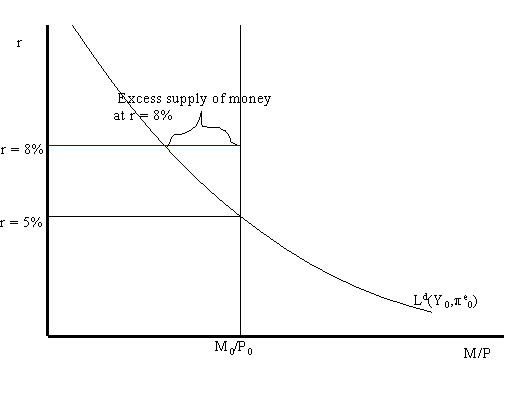# Equilibrium in the money market

Reading: AB, chapter 7, section 4.Real money demand and the real money supply as functions of the real interest rate are illustrated in the above graph. Real money demand is graphed holding fixed real income and expected inflation. The real money supply is equal to the nominal amount of M1, denoted M0, divided by the fixed aggregate price level, P0. It is assumed that the Fed does not alter the money supply based on the valued of the real interest rate. Therefore, the real money supply function is a vertical line in the graph with the real interest rate on the vertical axis and real money balances on the horizontal axis.

Notice that real money demand and real money supply intersect when the real interest rate is 5%. This is the value of the real interest that equates money demand with the money supply and establishes equilibrium in the money market. When the money market is in equilibrium there are no economic forces acting on the economy to alter the real interest rate.

If the real interest rate were 8% then the demand for real balances would be greater than the fixed supply of real balances (as illustrated above). In this case we say there is an excess supply of money in the money market. Practically, what this means is that individuals are holding more money than they would like given the high real interest rate. Accordingly, individuals will attempt to rebalance their portfilios; i.e. they will try to get rid of money by buying bonds (our generic non-money asset). In doing so the demand for bonds increases and so the price of bonds increases. Because bond prices are inversely related to the interest rate on bonds, the increased price of bonds lowers the real return on bonds (holding expected inflation fixed). Therefore, the excess supply of money at 8% (disequilibrium in the money market) leads to economic forces that act to lower the real interest rate. These forces cease to operate when the real interest falls to 5% where the demand for real balances is equal to the supply of real balances.

[Previous Slide] [Contents for Lecture 7] [Slides from Lectures] [301 Homepage]
Last updated on July 15, 1996 by Eric Zivot.